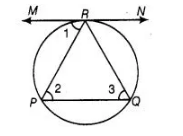# A chord PQ of a circle is parallel to

Question:

A chord PQ of a circle is parallel to the tangent drawn at a point R of the circle. Prove that R bisects the arc PRQ.

Solution:

Given Chord PQ is parallel to tangent at R.

To prove R bisects the arc PRQProof $\angle 1=\angle 2$ [alternate interior angles]

$\angle 1=\angle 3$

[angle between tangent and chord is equal to angle made by chord in alternate segment]

$\therefore$ $\angle 2=\angle 3$

$P R=Q R \quad$ sides opoosite to equal angles are equal

$\Rightarrow \quad P R=Q R$

So, $R$ bisects $P Q$.